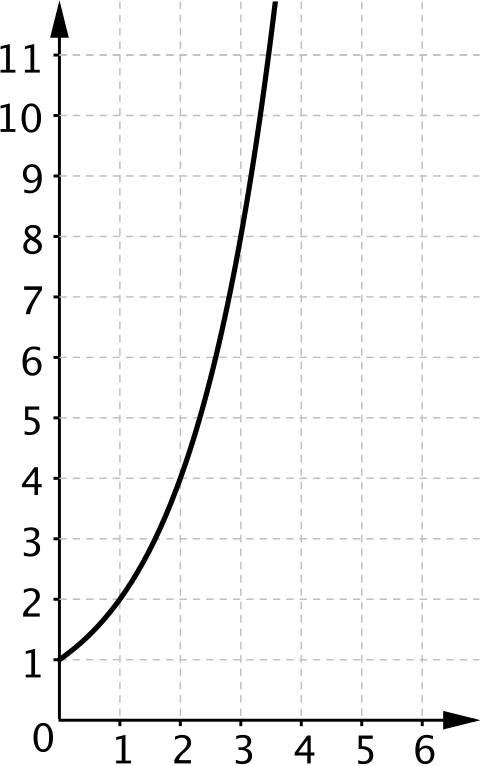#### You may also likeThis comes in two parts, with the first being less fiendish than the second. Itâ€™s great for practising both quadratics and laws of indices, and you can get a lot from making sure that you find all the solutions. For a real challenge (requiring a bit more knowledge), you could consider finding the complex solutions.### Discriminating

You're invited to decide whether statements about the number of solutions of a quadratic equation are always, sometimes or never true.This will encourage you to think about whether all quadratics can be factorised and to develop a better understanding of the effect that changing the coefficients has on the factorised form.

# Reach for the Stars

##### Age 16 to 18Challenge LevelThis resource is from Underground Mathematics.

Imagine plotting a graph of $y=2^x$, with $1$cm to one unit on each axis.How far along the $x$-axis could you go before the graph reached the top of a sheet of paper?

If you extended the graph so the positive $x$-axis filled the whole width of a sheet of paper, how tall would the paper have to be?

How far along the $x$-axis would you have to go so that the graph was tall enough to reach

• to the top of The Shard in London?
• to the moon?
• to the Andromeda galaxy?

Try to estimate the answers before calculating them and mark them at the appropriate points along a sketch of the $x$-axis.

Work out where they should be and then add some other results such as the distances to the sun and other stars. What do you notice?

We have provided some data below for you to work from, or you could research suitable data for yourself.

Paper sizes

*   A4 paper measures $298$mm by $210$mm.

*   A3 is double the area of A4 --- $298$mm by $420$mm.

*   To go from A4 to A3 and from A3 to A2 you double the shorter dimension each time. The sequence continues up to A0.

*   American Letter size paper is $8.5$in. by $11$in..

On Earth

*   The Shard in London is $309.6$m high. Its viewing gallery is approximately $244$m above ground level.

*   One of the tallest buildings on Earth is the Burj Khalifa in Dubai, at $829.8$m.

*   The highest mountain is Mount Everest, at $8\,848$m.

In astronomy

*   The mean distance from the Earth to the moon is $378\,000$km.

*   The mean distance from the Earth to the sun is $149\,600\,000$km.

*   After the Sun, the nearest bright star is Alpha Centauri, at a distance of about $4.4$light years from Earth.

One light year is the distance that light can travel in a year.  Light travels at a speed of about $300\,000$km/s.

*   Andromeda, the closest major galaxy to our own, is approximately $2.5$Mly from Earth.

$1$Mly (megalight year) is $10^6$light years.

*   The edge of the known Universe is approximately $13.8$ billion light years away.

This is an Underground Mathematics resource.

Underground Mathematics is hosted by Cambridge Mathematics. The project was originally funded by a grant from the UK Department for Education to provide free web-based resources that support the teaching and learning of post-16 mathematics.

Visit the site at undergroundmathematics.org to find more resources, which also offer suggestions, solutions and teacher notes to help with their use in the classroom.